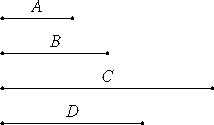# Proposition 2

If two numbers multiplied by one another make a square number, then they are similar plane numbers.

Let A and B be two numbers, and let A multiplied by B make the square number C.

I say that A and B are similar plane numbers.

Multiply A by itself to make D. Then D is square.VII.17

Now, since A multiplied by itself makes D, and multiplied by B makes C, therefore A is to B as D is to C.

And, since D is square, and C is so also, therefore D and C are similar plane numbers.

Therefore one mean proportional number falls between D and C. And D is to C as A is to B, therefore one mean proportional number falls between A and B also.

VIII.20

But, if one mean proportional number falls between two numbers, then they are similar plane numbers, therefore A and B are similar plane numbers.

Therefore, if two numbers multiplied by one another make a square number, then they are similar plane numbers.

Q.E.D.

## Guide

This proposition is a converse of the previous one.

As an example to illustrate this proposition, take any square number, such as 202 = 400. It can be factored as a product of two numbers in several ways. One such factorization is as a = 50 times b = 8. These two numbers have a mean proportional between them, namely, 20, so by VIII.20, they are similar plane numbers. (The actual shapes given by that proposition make 8 to be 2 by 4, and 50 to be 5 by 10.)

#### Outline of the proof

Let a and b be two numbers whose product ab is a square. Now, both a2 and ab are square numbers which means that they’re similar plane numbers. By VIII.8, there’s a mean proportional between them. But a2 : ab = a : b, so there is also a mean proportional between a and b (VIII.8). Therefore, a and b are similar plane figures (VIII.20).

As in the last proof, this one can be shortened. When the product ab is a square, say e2, then a mean proportional between a and b is e.